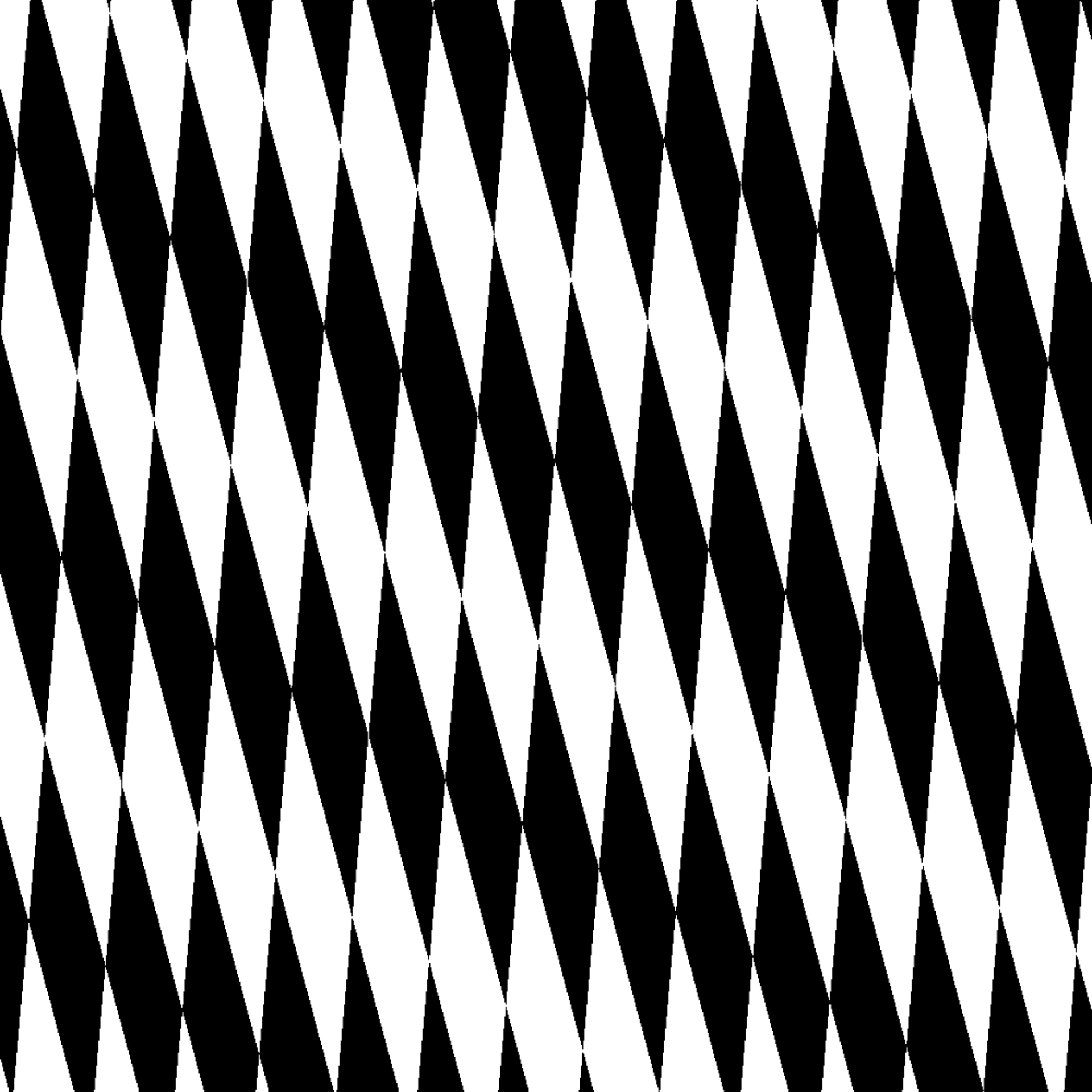This function allows you to calculate linear transformations of coordinates in a long_grid object. You can either pass in a transformation matrix or a trans object as produced by ggforce::linear_trans(...). The latter makes it easy to stack multiple transformations into one, but require the ggforce package.

trans_affine(x, y, ...)

rotate(angle = 0)

stretch(x0 = 0, y0 = 0)

shear(x0 = 0, y0 = 0)

translate(x0 = 0, y0 = 0)

reflect(x0 = 0, y0 = 0)

## Arguments

x, y The coordinates to transform A sequence of transformations An angle in radians the transformation magnitude in the x-direction the transformation magnitude in the x-direction

## Linear Transformations

The following transformation matrix constructors are supplied, but you can also provide your own 3x3 matrices to translate()

• rotate(): Rotate coordinates by angle (in radians) around the center counter-clockwise.

• stretch(): Stretches the x and/or y dimension by multiplying it with x0/y0.

• shear(): Shears the x and/or y dimension by x0/y0.

• translate(): Moves coordinates by x0/y0.

• reflect(): Reflects coordinates through the line that goes through 0, 0 and x0, y0.

## Examples

grid <- long_grid(seq(1, 10, length.out = 1000), seq(1, 10, length.out = 1000))
grid$trans <- trans_affine(grid$x, grid$y, rotate(pi/3), shear(-2), rotate(-pi/3)) grid$chess <- gen_checkerboard(grid$trans$x, grid$trans$y)

plot(grid, chess)